Logarithmic Differentiation - Type 2

Chapter 5 Class 12 Continuity and Differentiability
Concept wise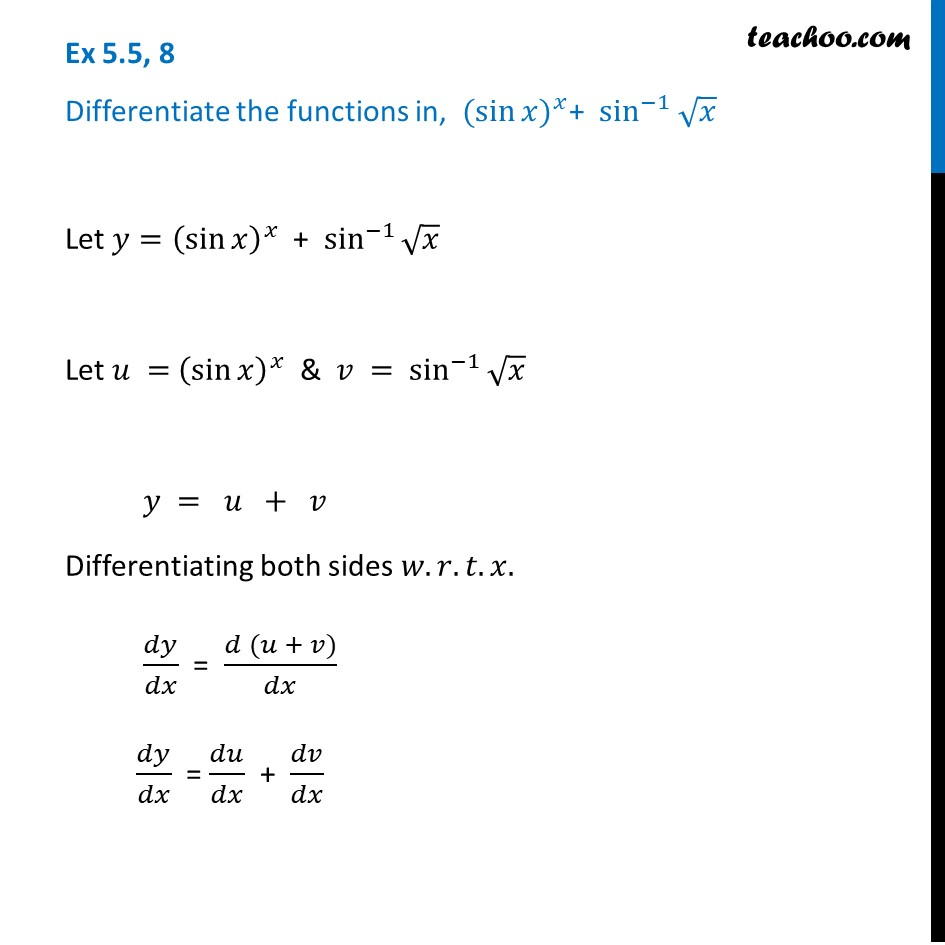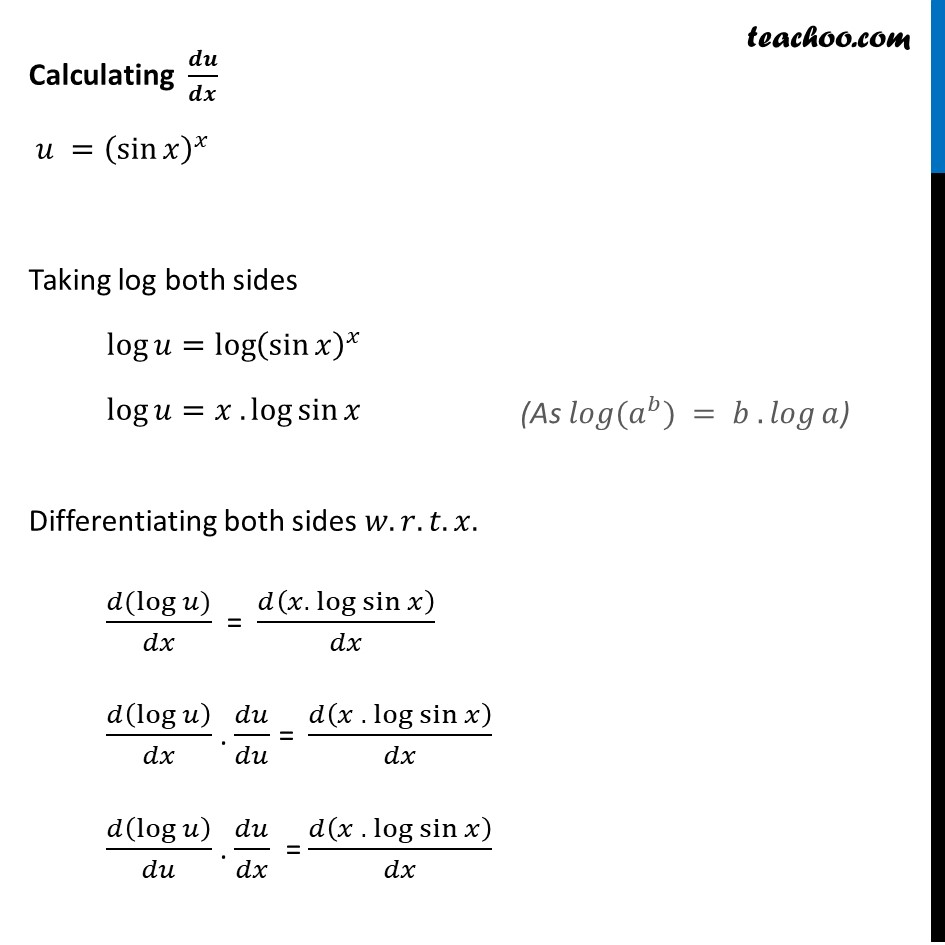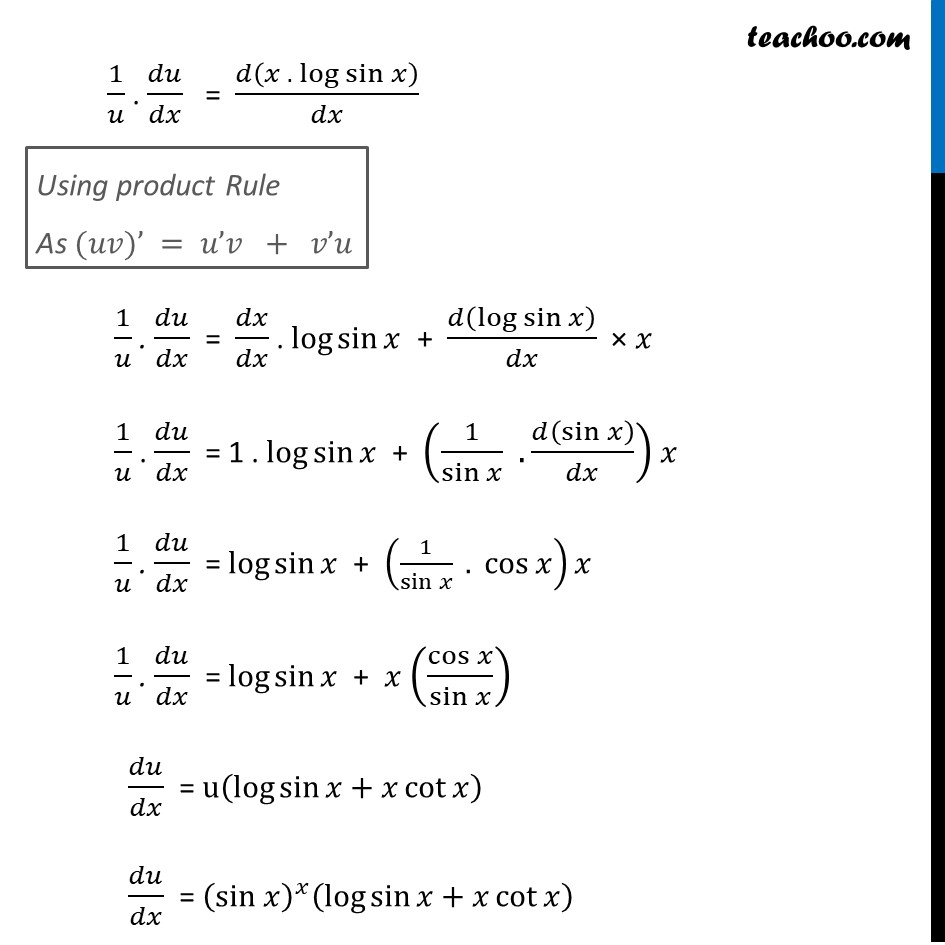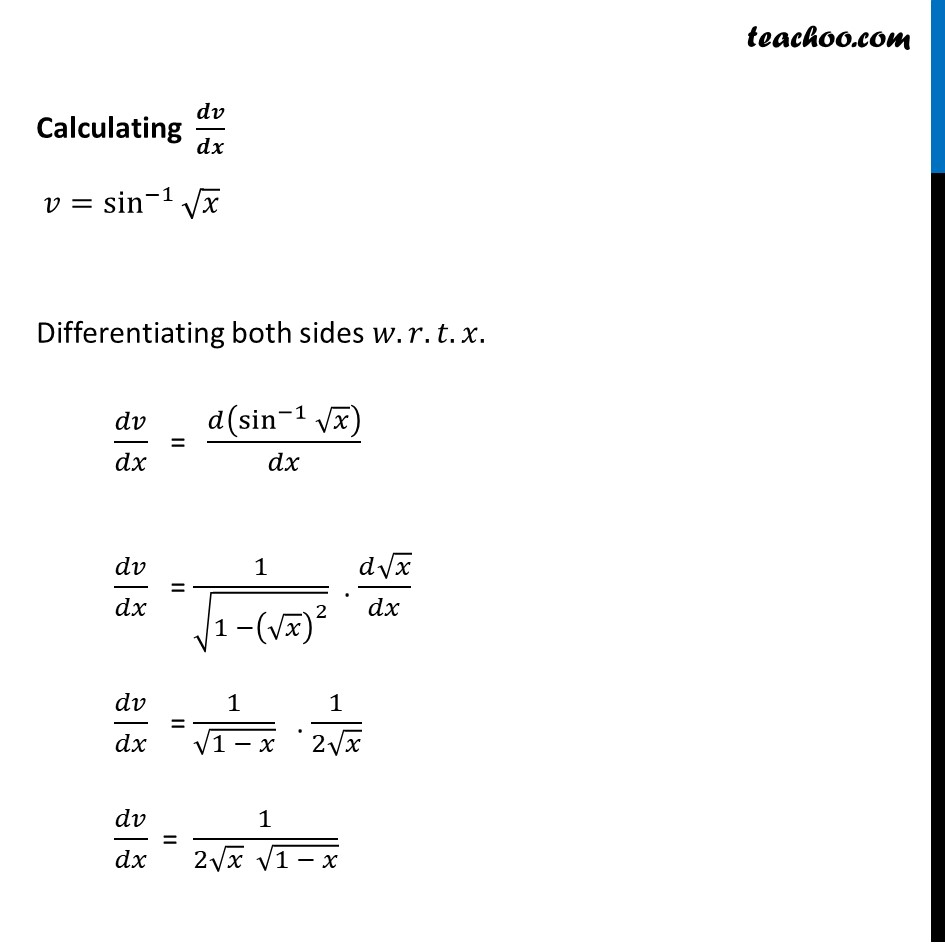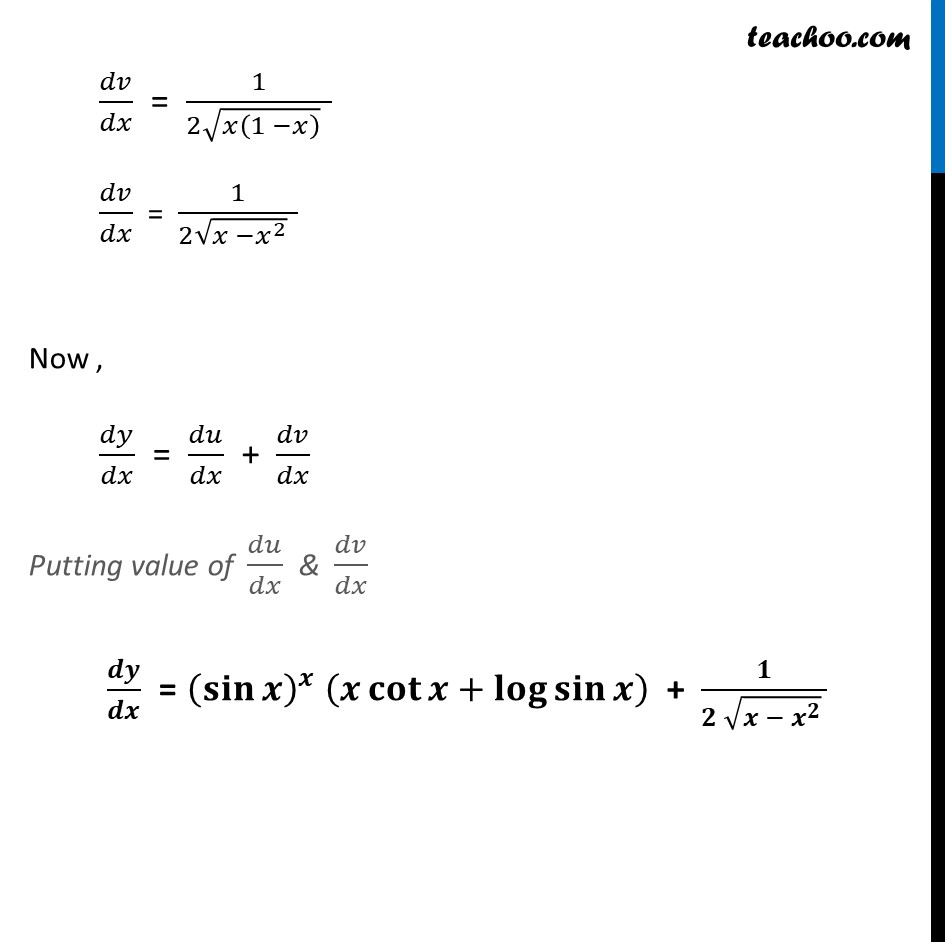Introducing your new favourite teacher - Teachoo Black, at only ₹83 per month

### Transcript

Ex 5.5, 8 Differentiate the functions in, 〖(sin⁡𝑥)〗^𝑥+ sin^(−1) √𝑥 Let 𝑦=(sin⁡𝑥 )^𝑥 + sin^(−1)⁡√𝑥 Let 𝑢 = (sin⁡𝑥 )^𝑥 & 𝑣 = sin^(−1)⁡√𝑥 𝑦 = 𝑢 + 𝑣 Differentiating both sides 𝑤.𝑟.𝑡.𝑥. 𝑑𝑦/𝑑𝑥 = (𝑑 (𝑢 + 𝑣))/𝑑𝑥 𝑑𝑦/𝑑𝑥 = 𝑑𝑢/𝑑𝑥 + 𝑑𝑣/𝑑𝑥 Calculating 𝒅𝒖/𝒅𝒙 𝑢 =(sin⁡𝑥 )^𝑥 Taking log both sides log⁡𝑢=log⁡〖(sin⁡𝑥 )^𝑥 〗 log⁡𝑢=𝑥 .log⁡sin⁡〖𝑥 〗 Differentiating both sides 𝑤.𝑟.𝑡.𝑥. (𝑑(log⁡𝑢))/𝑑𝑥 = 𝑑(𝑥.〖 log〗⁡sin⁡𝑥 )/𝑑𝑥 𝑑(log⁡𝑢 )/𝑑𝑥 . 𝑑𝑢/𝑑𝑢 = 𝑑(𝑥 .〖 log〗⁡sin⁡𝑥 )/𝑑𝑥 𝑑(log⁡𝑢 )/𝑑𝑢 . 𝑑𝑢/𝑑𝑥 = 𝑑(𝑥 .〖 log〗⁡sin⁡𝑥 )/𝑑𝑥 (As 𝑙𝑜𝑔⁡(𝑎^𝑏) = 𝑏 . 𝑙𝑜𝑔⁡𝑎) 1/𝑢 . 𝑑𝑢/𝑑𝑥 = 𝑑(𝑥 .〖 log〗⁡sin⁡𝑥 )/𝑑𝑥 1/𝑢 . 𝑑𝑢/𝑑𝑥 = 𝑑𝑥/𝑑𝑥 . log⁡sin⁡𝑥 + 𝑑(log⁡sin⁡𝑥 )/𝑑𝑥 × 𝑥 1/𝑢 . 𝑑𝑢/𝑑𝑥 = 1 . log⁡sin⁡𝑥 + (1/sin⁡𝑥 . 𝑑(sin⁡𝑥 )/𝑑𝑥) 𝑥 1/𝑢 . 𝑑𝑢/𝑑𝑥 = log⁡sin⁡𝑥 + (1/sin⁡𝑥 . cos⁡𝑥 )𝑥 1/𝑢 . 𝑑𝑢/𝑑𝑥 = log⁡sin⁡𝑥 + 𝑥 (cos⁡𝑥/sin⁡𝑥 ) 𝑑𝑢/𝑑𝑥 = u(log⁡sin⁡〖𝑥+𝑥 cot⁡𝑥 〗 ) 𝑑𝑢/𝑑𝑥 = (sin 𝑥)^𝑥 (log⁡sin⁡〖𝑥+𝑥 cot⁡𝑥 〗 ) Using product Rule As (𝑢𝑣)’ = 𝑢’𝑣 + 𝑣’𝑢 Calculating 𝒅𝒗/𝒅𝒙 𝑣=sin^(−1)⁡√𝑥 Differentiating both sides 𝑤.𝑟.𝑡.𝑥. 𝑑𝑣/𝑑𝑥 = 𝑑(sin^(−1)⁡√𝑥 )/𝑑𝑥 𝑑𝑣/𝑑𝑥 = 1/√(1 −(√𝑥)^2 ) . (𝑑√𝑥)/𝑑𝑥 𝑑𝑣/𝑑𝑥 = 1/√(1 − 𝑥) . 1/(2√𝑥) 𝑑𝑣/𝑑𝑥 = 1/(2√𝑥 √(1 − 𝑥)) 𝑑𝑣/𝑑𝑥 = 1/(2√(𝑥(1 −𝑥) ) ) 𝑑𝑣/𝑑𝑥 = 1/(2√(𝑥 −𝑥^2 ) ) Now , 𝑑𝑦/𝑑𝑥 = 𝑑𝑢/𝑑𝑥 + 𝑑𝑣/𝑑𝑥 Putting value of 𝑑𝑢/𝑑𝑥 & 𝑑𝑣/𝑑𝑥 𝒅𝒚/𝒅𝒙 = (𝐬𝐢𝐧⁡𝒙 )^𝒙 (𝒙 𝐜𝐨𝐭⁡𝒙+𝐥𝐨𝐠⁡𝐬𝐢𝐧⁡𝒙 ) + 𝟏/(𝟐 √(𝒙 − 𝒙^𝟐 ) )